# Cell ranges: basic things 99% of users don’t know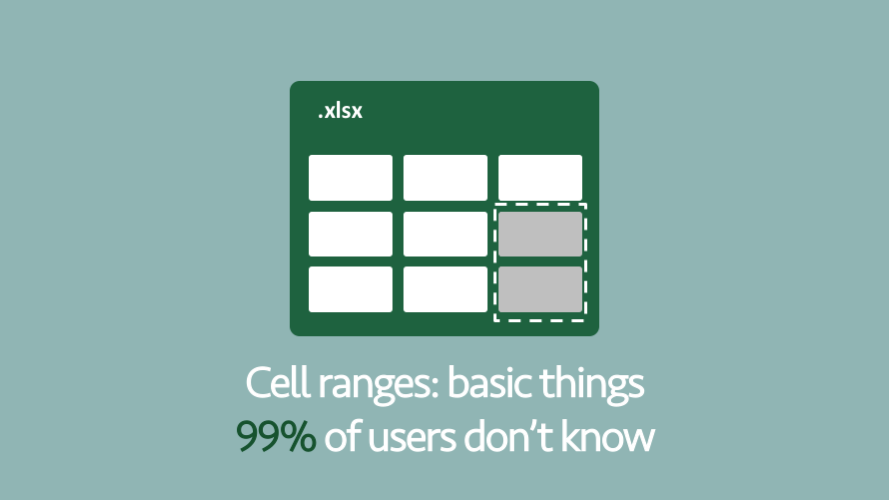I have never been taught how to use ranges in Excel, I doubt you have either.  They are just too basic for anybody to teach, right?  Select some cells and there is a : (colon) symbol placed between the start cell and the end cell.  Maybe we could throw in a few \$ (dollar signs) to fix the position of a cell reference.  It’s hardly rocket science.

For most Excel users that is everything they know about cell ranges, and everything they think they need to know about cell ranges.  But that’s just the start point – there is so much more.  In this post, we will uncover a few other basic methods for using ranges.  Every Excel user should know these (but most of them don’t).

We will be considering the following:

• Range operator – Referencing cells in a continuous range
• Union operator – Joining ranges together
• Intersection operator – The overlapping cells of two ranges
• Functions which return cell ranges

## Range operator

The Range operator is the : (colon) symbol.  This is the part which most Excel users do know.  So, I won’t spend too long on this.  Here is an example of the Range operator being used.

`B2:D4`

The Range Operator combines all the cells (including the cells being referenced) into a single reference.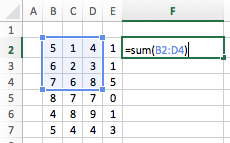Nothing too complex here.  But once joined with the Union operator or the Intersection operator it is ever more powerful.

## Union operator

The Union operator is the , (comma) symbol.  Most Excel users have used this at some point, but are not really aware that it can be combined with the Range operator.  You may have seen the Union operator it in the following situation:From the screenshot, it appears that we are simply listing cells with a comma separating them.  We understand this concept because that’s how we list items in a sentence, but that’s not how cell references work.  We are actually combining the cells together into a single range.

The power of the Union operator really comes when combined with the Range operator.  Look at the image below, we are summing the values in the two ranges as if there were just a single range.But there is even more power to be found when using the Union operator in a “what-if” type scenario.  Look at the image below, we are using the AVERAGE function to find the average value from the Range B2-B7.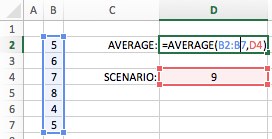By including Cell D4 into the formula we are answering the question, “What would the average be if the next value were 9?”.  A simple example, but very useful.

## Intersection operator

The Intersection operator is the (space) character.  Very few Excel users are aware of this feature.  Look at the example below: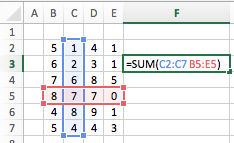The formula in Cell F3 will result in a value of 7.  The two ranges intersect (the area where the ranges cross over) at cell C5, so the value returned in in Cell F3 is equal to Cell C5.

When applying this to named ranges we can create a simple lookup formula without the need of any functions.

In the example below, I have added named ranges to each column based on the values in Row 1 and to each row based on the values in Column A. I can now use the Intersection operator to create a lookup based on the Month and the Region. The value returned in Cell F2 below would be 7.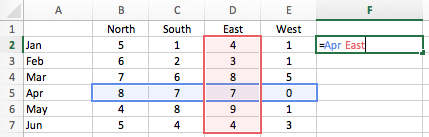Going even further, we can sum the Intersection ranges together.  In the screenshot below the value returned in Cell F2 would be 11 (8+3).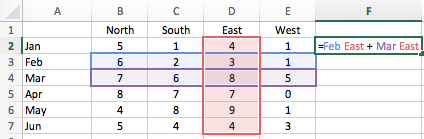It is also possible to use the Intersection operator with the Range operator, look at the following example:Based on this formula, the value in Cell F3 will become 5 (2 = Feb South + 3 = Feb East).

Formula Magic with Dynamic ArraysOf all the features available in Excel dynamic arrays provide the most power for the smallest time investment.  Yet most Excel users do not even know what they are.

Have you ever faced these spreadsheet scenarios?

• How can I use VLOOKUP to return all the matching items, not just the first?
• How can I sort my information using a formula, so I don’t have to keep clicking the sort button?
• How can I quickly create unique lists of items to use with my SUMIFS calculation?
• How can I stop copying down formulas every time my source data changes.
• How can I build a PivotTable-like report, but using formulas so I don’t have to click refresh ever again.

Well, I’m here to give you some good news. with dynamic arrays, all these can be achieved easily 🙂

## Functions which return cell ranges

One of the challenges of working with ranges, is when we don’t know the address of the cells to include in the range.  Thankfully there are a number of functions we can use, which return the address of the cell.

### INDIRECT

INDIRECT takes a text string and converts it into a range.  For example:

`=INDIRECT("A"&F3)`

The formula above would take the value in Cell F3 as being the row number.  So, if Cell F3 contained a 5, the Cell Reference would be A5.

INDIRECT is a volatile function, so should be used with care.

### OFFSET

The OFFSET function can have up to 5 arguments.

• Reference: is the cell from which you want to base the offset
• Rows: is the number of rows, that you want the Reference cell to move by.
• Cols: is the number of columns, that you want the Reference cell to move by.
• Height (optional): is number of rows you want the range to be
• Width (optional): is the number of columns you want the range to be

It might be easier to understand with an example.  Look at the formula in Cell F3.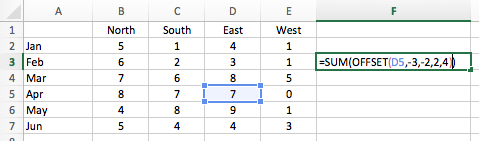The OFFSET starts with the Reference Cell (Cell D5), moves -3 rows (up to Cell D2), then -2 columns (across to Cell B2).  The last two arguments calculate the size of the new range.  So, starting at Cell B2, the height is 2 and the width is 4.  Therefore, the final range is B2:E3.

The result of the formula in Cell F3 is 23 (which is the Sum of range B2:E3).

OFFSET is a volatile function, so should be used with care.

### INDEX

INDEX is similar to the first 3 arguments of the OFFSET function.  The key differences being (1) the first argument must be the range of cells which includes the result cell (2) due to the nature of the function, the counting starts at 1 (e.g. INDEX(A1:C3,1,1) is the same cell reference as OFFSET(A1,0,0)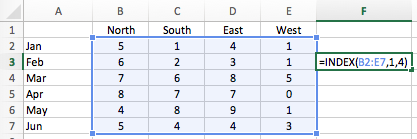It is now possible to use INDEX in unique ways.  Look at the screenshot below.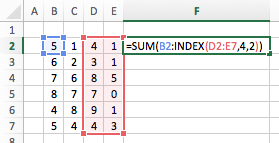Does the formula in Cell F2 make any sense to you?

`=SUM(B2:INDEX(D2:E7,4,2))`

Looking first at the INDEX function. In the Range D2-E7 take the 4th row and 2nd column, the result of which is E5.  Therefore, the formula becomes:

`=SUM(B2:E5)`

That’s pretty neat, right?

INDEX is not a volatile function, and therefore should be used ahead of OFFSET and INDIRECT where possible.

### CHOOSE

The CHOOSE function can be used to select different cell ranges based on an index number.  Here is an example:

`=SUM(CHOOSE(2,B2:B7,C2:C7,D2:D7,E2:E7))`

The first argument of the CHOOSE function is the index number.  The following arguments are a list of possible results.  Using the example above, the first item in the list is B2:B7, the second is C2:C7, the third is D2:D7 and so on.

As the index number provided in the example above is 2, the result of the CHOOSE function will be the 2nd item in the list – Cells C2:C7.  (Remember, it will be the third argument, as the first argument in the function is not part of the list).

The formula above becomes:

`=SUM(C2:C7)`

### SWITCH

The SWITCH function (Excel 2016 onwards) operates in a similar way to CHOOSE.  However, rather than selecting an item based on an index it will return a result based on a matched value.

`=SUM(SWITCH(F2,"North",B2:B7,"South",C2:C7,"East",D2:D7,"West",E2:E7))`

Using the example above, if the value in Cell F2 is “East”, the Cells D2:D7 will be returned by the SWITCH function.  The formula above becomes:

`=SUM(D2:D7)`

## Conclusion

You’re now an Excel Range ninja.  You’ve got all the tools you need to create some very powerful (yet simple) Excel models.  The only limit now, is your creativity.Get our FREE VBA eBook of the 30 most useful Excel VBA macros.
Automate Excel so that you can save time and stop doing the jobs a trained monkey could do.

Email Address * First Name *

Don’t forget:

I’m guessing the examples in this post didn’t exactly meet your situation.  We all use Excel differently, so it’s impossible to write a post that will meet everybody’s needs.  By taking the time to understand the techniques and principles in this post (and elsewhere on this site) you should be able to adapt it to your needs.

But, if you’re still struggling you should:

1. Read other blogs, or watch YouTube videos on the same topic.  You will benefit much more by discovering your own solutions.
2. Ask the ‘Excel Ninja’ in your office.  It’s amazing what things other people know.
3. Ask a question in a forum like Mr Excel, or the Microsoft Answers Community.  Remember, the people on these forums are generally giving their time for free.  So take care to craft your question, make sure it’s clear and concise.  List all the things you’ve tried, and provide screenshots, code segments and example workbooks.
4. Use Excel Rescue, who are my consultancy partner.   They help by providing solutions to smaller Excel problems.

What next?
Don’t go yet, there is plenty more to learn on Excel Off The Grid.  Check out the latest posts:

Save

## 4 thoughts on “Cell ranges: basic things 99% of users don’t know”

1.David Hager says:

And the CHOOSE function.

•Excel Off The Grid says:

Hi David,

Ah, yes. Good call. The new SWITCH function can also return a range. I’ll update the article to include both of these.

Can you think of any others I’ve missed?

2.Martin Klima says:

Well, I really wish it was true, but it isn’t. All your examples work only because the formulas you show accept multiple ranges and comma is just an argument separator. If you try this approach with a function that only accepts one range (e.g. UNIQUE), you will get an error.

•Excel Off The Grid says:

Yes, UNIQUE will give you an error if used with some of these techniques; it’s intended to work with non-contiguous ranges. It doesn’t negate the features of ranges but shows the functions are designed to work with specific inputs.